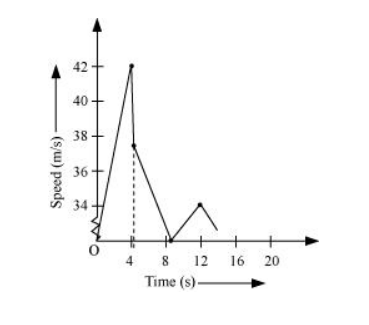# A ball is dropped from a height of 90 m on a floor.

Question.
A ball is dropped from a height of $90 \mathrm{~m}$ on a floor. At each collision with the floor, the ball loses one tenth of its speed. Plot the speed-time graph of its motion between $t=0$ to $12 \mathrm{~s} .$

solution:

Ball is dropped from a height, s = 90 m

Initial velocity of the ball, $u=0$

Acceleration, $a=g=9.8 \mathrm{~m} / \mathrm{s}^{2}$

Final velocity of the ball $=v$

From second equation of motion, time $(t)$ taken by the ball to hit the ground can be obtained as:

$s=u t+\frac{1}{2} a t^{2}$

$90=0+\frac{1}{2} \times 9.8 t^{2}$

$t=\sqrt{18.38}=4.29 \mathrm{~s}$

From first equation of motion, final velocity is given as:

$v=u+a t$

$=0+9.8 \times 4.29=42.04 \mathrm{~m} / \mathrm{s}$

Rebound velocity of the ball, $u_{\mathrm{r}}=\frac{9}{10} v=\frac{9}{10} \times 42.04=37.84 \mathrm{~m} / \mathrm{s}$

Time $(t)$ taken by the ball to reach maximum height is obtained with the help of first equation of motion as:

$v=u_{r}+a t^{\prime}$

$0=37.84+(-9.8) t$

$t^{\prime}=\frac{-37.84}{-9.8}=3.86 \mathrm{~s}$

Total time taken by the ball $=t+t^{\prime}=4.29+3.86=8.15 \mathrm{~s}$

As the time of ascent is equal to the time of descent, the ball takes $3.86 \mathrm{~s}$ to strike back on the floor for the second time.

The velocity with which the ball rebounds from the floor $=\frac{9}{10} \times 37.84=34.05 \mathrm{~m} / \mathrm{s}$

Total time taken by the ball for second rebound $=8.15+3.86=12.01 \mathrm{~s}$

The speed-time graph of the ball is represented in the given figure as: